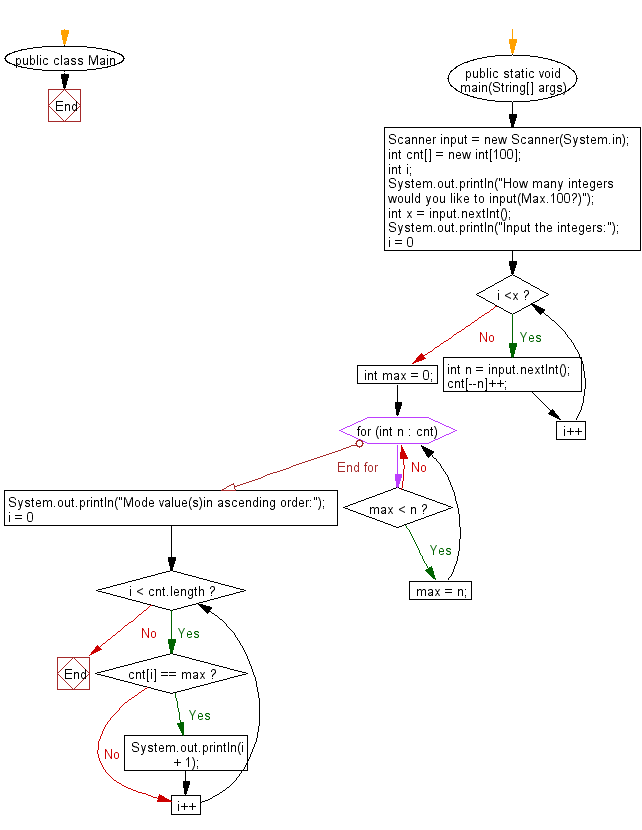# Java Exercises: Print mode values from a given a sequence of integers

## Java Basic: Exercise-226 with Solution

Write a Java program to print mode values from a given a sequence of integers. The mode value is the element which occurs most frequently. If there are several mode values, print them in ascending order.

Input:

A sequence of integer’s ai (1 ≤ ai ≤ 100). The number of integers is less than or equals to 100.

Sample Solution:

Java Code:

``````import java.util.Scanner;
public class Main {
public static void main(String[] args){
Scanner input = new Scanner(System.in);
int cnt[] = new int;
int i;
System.out.println("How many integers would you like to input(Max.100?)");
int x = input.nextInt();
System.out.println("Input the integers:");
for (i = 0; i <x; i++){
int n = input.nextInt();
cnt[--n]++;
}

int max = 0;
for (int n : cnt){
if (max < n){
max = n;
}
}
System.out.println("Mode value(s)in ascending order:");
for (i = 0; i < cnt.length; i++){
if (cnt[i] == max){
System.out.println(i + 1);
}
}
}
}
```
```

Sample Output:

```How many integers would you like to input(Max.100?)
5
Input the integers:
25
35
15
5
45
Mode value(s)in ascending order:
5
15
25
35
45
```

Pictorial Presentation:Flowchart:Java Code Editor:

What is the difficulty level of this exercise?

﻿

## Java: Tips of the Day

Parsing dates:

```import java.io.*;
import java.util.*;
import java.text.*;

String s = "2001/09/23 14:39";

SimpleDateFormat formatter = new SimpleDateFormat ("yyyy/MM/dd H:mm");
Date d = formatter.parse(s, new ParsePosition(0));
```